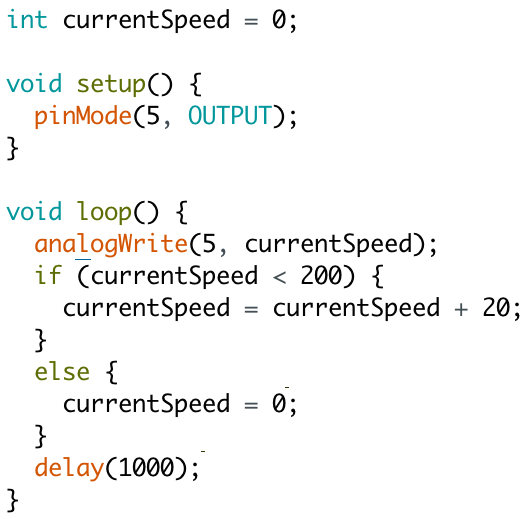# Fun With Conditionals and Control Structures

Conditionals are statements that return either true or false. For example, the conditional statement 100 > 70 evaluates as true, whereas 0 > 300 is false.

Some different kinds of conditional statements we can make are:

• x < y (x is less than y)
• x > y (x is greater than y)
• x == y (x is equal to y)

Control structures use conditionals to make choices within code. One type of control structure is if and else statements.In this program, we're keeping track of the vibrator motor's current speed in a variable that we set before the setup and loop start (currentSpeed). Until the vibration is at 200 intensity, we increase it by 20 every second. At 200 intensity, it's reset back to 0.This is a for loop. This program does the exact same thing as the last program, only it says it in a slightly different way. A for loop has 3 parts: an initialization step, a conditional, and an after effect. The initialization step is run at the beginning of the for loop, in this case setting the variable "x" to 0. While the condition x < 10 is true, the loop will repeat. At the end of each iteration of the loop, the after effect (x++, the same thing as x = x + 1) will run. You can use a for loop to repeat a series of instructions a set number of times.

## Exercises

• Copy the program shown above and test it out on your vibrator.
• Right now the program starts at 0 intensity and increases to 200 intensity. How would we make it start at 200 intensity and gradually fade to 0 intensity?
• Hmm, this program might be too intense. How can we add a 2000 millisecond pause whenever the vibration speed is at 0?
• The pause is nice, but it would also be good for the highest speed vibration to last longer. How can we make the program vibrate at the highest speed for 3000 milliseconds before fading out?
• Let's make the program more interesting. How can we make a fade in (so 0 intensity, then 20 intensity, then 40 intensity, etc.) following the fade out?